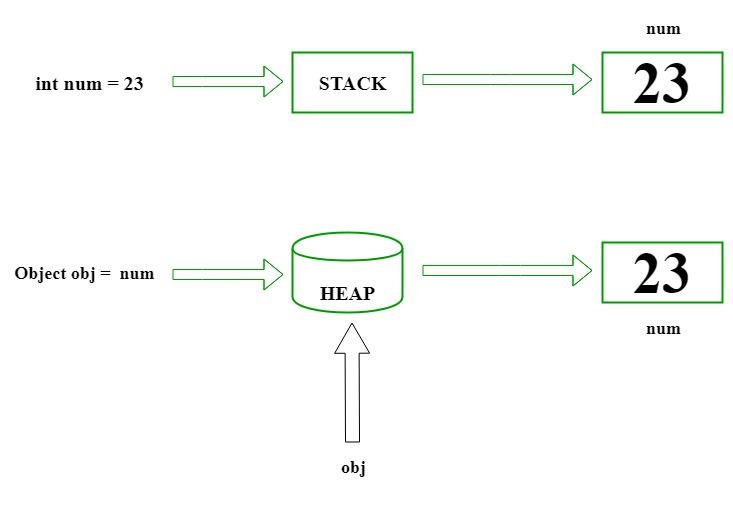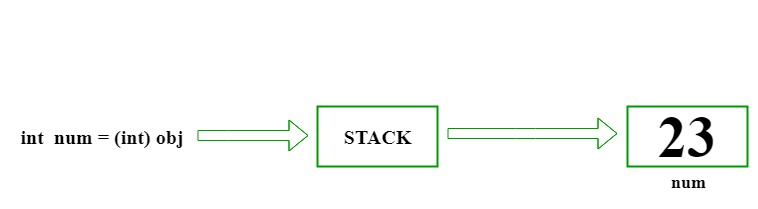Related Articles
C# | Boxing And Unboxing
• Difficulty Level : Easy
• Last Updated : 30 Jul, 2018

#### Prerequisite : Data Types in C#

Boxing and unboxing is an important concept in C#. C# Type System contains three data types: Value Types (int, char, etc), Reference Types (object) and Pointer Types. Basically it convert a Value Type to a Reference Type, and vice versa. Boxing and Unboxing enables a unified view of the type system in which a value of any type can be treated as an object.

### Boxing In C#

• The process of Converting a Value Type (char, int etc.) to a Reference Type(object) is called Boxing.
• Boxing is implicit conversion process in which object type (super type) is used.
• The Value type is always stored in Stack. The Referenced Type is stored in Heap.
• Example :
```int num = 23; // 23 will assigned to num
Object Obj = num; // Boxing
```
• Description : First declare a value type variable (num), which is integer type and assigned it with value 23. Now create a references object type (obj) and applied Explicit operation which results in num value type to be copied and stored in object reference type obj as shown in below figure :• Let’s understand Boxing with a C# programming code :
 `// C# implementation to demonstrate``// the Boxing``using` `System;``class` `GFG {`` ` `    ``// Main Method``    ``static` `public` `void` `Main()``    ``{`` ` `        ``// assigned int value``        ``// 2020 to num``        ``int` `num = 2020;`` ` `        ``// boxing``        ``object` `obj = num;`` ` `        ``// value of num to be change``        ``num = 100;`` ` `        ``System.Console.WriteLine``        ``(``"Value - type value of num is : {0}"``, num);``        ``System.Console.WriteLine``        ``(``"Object - type value of obj is : {0}"``, obj);``    ``}``}`
Output:

```Value - type value of num is : 100
Object - type value of obj is : 2020
```

### Unboxing In C#

• The process of converting reference type into the value type is known as Unboxing.
• It is explicit conversion process.
• Example :
```int num = 23;         // value type is int and assigned value 23
Object Obj = num;    // Boxing
int i = (int)Obj;    // Unboxing
```
• Description : Declaration a value type variable (num), which is integer type and assigned with integer value 23. Now, create a reference object type (obj).The explicit operation for boxing create an value type integer i and applied casting method. Then the referenced type residing on Heap is copy to stack as shown in below figure :• Let’s understand Unboxing with a C# programming code :
 `// C# implementation to demonstrate``// the Unboxing``using` `System;``class` `GFG {`` ` `    ``// Main Method``    ``static` `public` `void` `Main()``    ``{`` ` `        ``// assigned int value``        ``// 23 to num``        ``int` `num = 23;`` ` `        ``// boxing``        ``object` `obj = num;`` ` `        ``// unboxing``        ``int` `i = (``int``)obj;`` ` `        ``// Display result``        ``Console.WriteLine(``"Value of ob object is : "` `+ obj);``        ``Console.WriteLine(``"Value of i is : "` `+ i);``    ``}``}`
Output:
```Value of ob object is : 23
Value of i is : 23
```

Attention reader! Don’t stop learning now. Get hold of all the important DSA concepts with the DSA Self Paced Course at a student-friendly price and become industry ready.

My Personal Notes arrow_drop_up